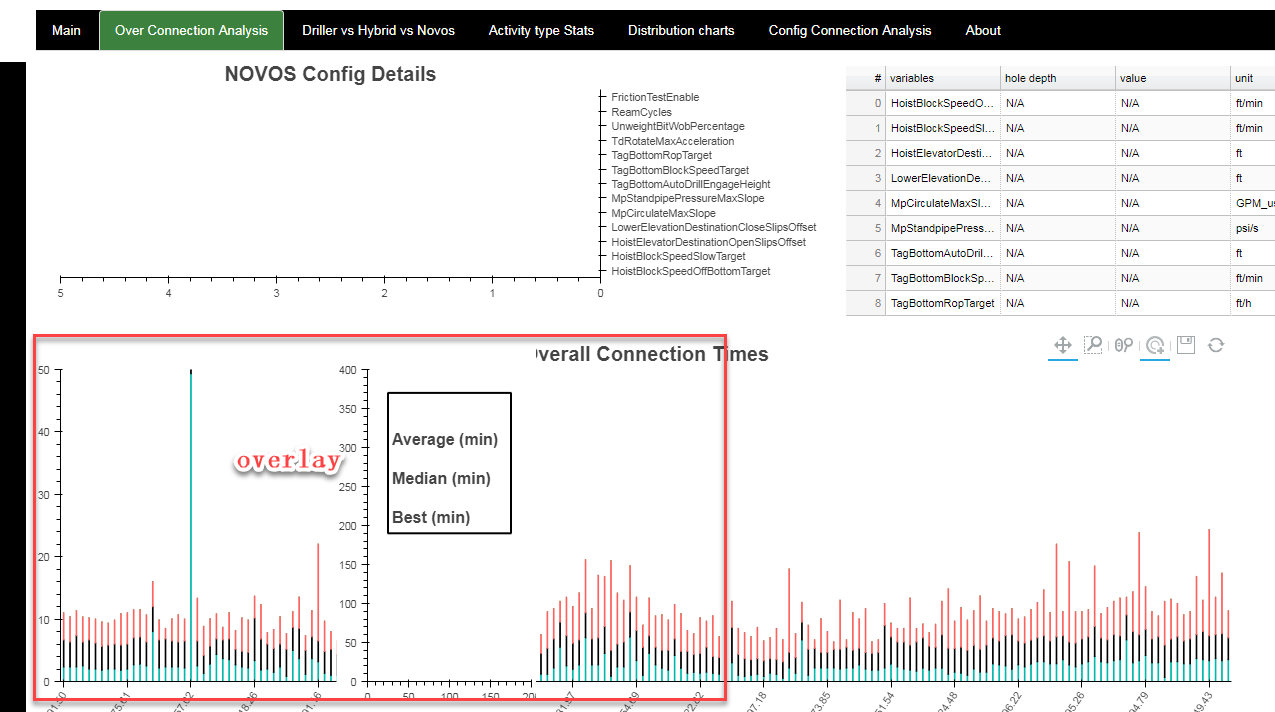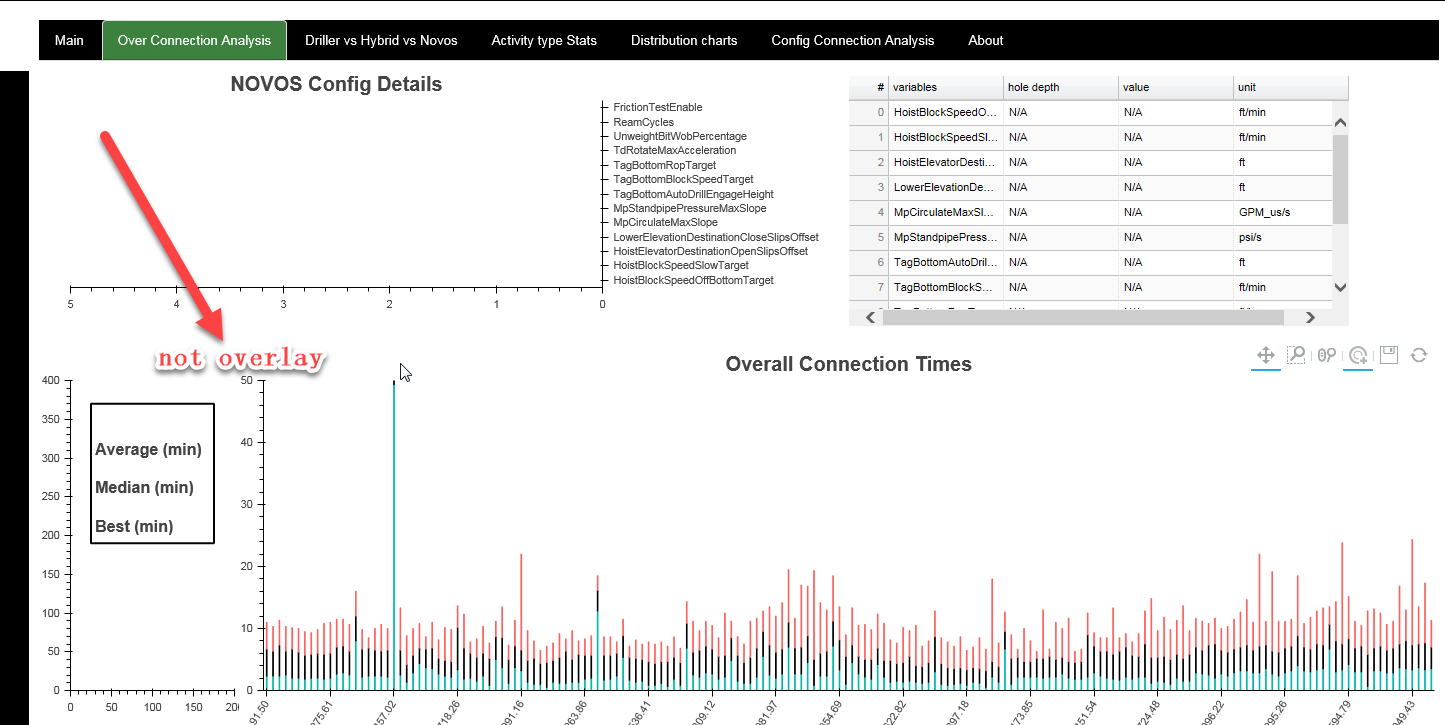# layout issue

I have an issue with layout, I largely know this is a bokeh bug,

I use bokeh 0.13.0, and crated a tab, and then

main_plot = figure(x_range = FactorRange(), \

y_range = (0, 50), \

plot_width = plot_width, \

plot_height = 400, \

tools = “tap, wheel_zoom, save, pan, box_zoom, reset”, \

sizing_mode = ‘scale_width’)

uHelper.main_plot.x_range.factors =

``

and then

main_textbox_plot = figure(x_range = (0, 200), \

y_range = (0, 400), \

plot_width = 200, \

plot_height = 400, \

sizing_mode = ‘scale_width’)

``

and thenmain_plot_row = row(main_plot, main_textbox_plot)
over_conn_analysis_main = column(novos_config_row, main_plot_row)

``

if I do something like thismain_plot_row = row( main_textbox_plot, main_plot,)

``

thanks for any feedback.

Hi,

I have an issue with layout, I largely know this is a bokeh bug,

I use bokeh 0.13.0, and crated a tab, and then

main_plot = figure(x_range = FactorRange(), \

y_range = (0, 50), \

plot_width = plot_width, \

plot_height = 400, \

tools = “tap, wheel_zoom, save, pan, box_zoom, reset”, \

sizing_mode = ‘scale_width’)

uHelper.main_plot.x_range.factors =

``

and then

main_textbox_plot = figure(x_range = (0, 200), \

y_range = (0, 400), \

plot_width = 200, \

plot_height = 400, \

sizing_mode = ‘scale_width’)

``

and thenmain_plot_row = row(main_plot, main_textbox_plot)
over_conn_analysis_main = column(novos_config_row, main_plot_row)

``

if I do something like thismain_plot_row = row( main_textbox_plot, main_plot,)

``

thanks for any feedback.

this is almost certainly a bug, but to discuss layout, you have to provide an entire layout scheme you’re using (novos_config_row in particular), because seemingly unrelated things may influence layout’s behavior significantly. Anyway, existing layout is just broken and will be replaced in bokeh 1.1.

Mateusz

···

On Wed, Nov 14, 2018 at 10:12 PM peng wang [email protected] wrote:

You received this message because you are subscribed to the Google Groups “Bokeh Discussion - Public” group.

To unsubscribe from this group and stop receiving emails from it, send an email to [email protected].

To post to this group, send email to [email protected].

To view this discussion on the web visit https://groups.google.com/a/continuum.io/d/msgid/bokeh/3cb6aaa8-96fa-4a5a-97b8-61028ebd8e5e%40continuum.io.